# College Physics with Mastering Physics

Physics & Astronomy

## Quiz 13 : Vibrations and WavesStudy FlashcardsLooking for Introductory Physics Homework Help?

## Quiz 13 :Vibrations and Waves

Question TypeA particle in simple harmonic motion has a frequency of 40 Hz. What is the period of this oscillation
Free
Essay

Time period is defined as the time taken for one complete oscillation. It is equal to the inverse of the frequency of the oscillation.
The relationship between time period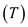and frequency of oscillation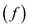is,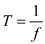Substitute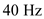for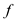in equation.Therefore, the period of oscillation is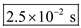.

Tags
Choose question tagIf the amplitude of a particle in SHM is doubled, how are (a) the total energy and (b) the maximum speed affected
Free
Essay

(a)
The expression for the total energy of the particle executing Simple harmonic motion is,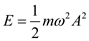Here, m is the mass of the particle,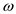is the angular frequency of oscillation, and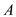is the amplitude of the particle.
From the above expression, keeping the other parameters constant, it is clear that the total energy of the particle in the simple harmonic motion is directly proportional to the square of the amplitude of the particle.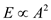Therefore, if the amplitude of a particle in the simple harmonic motion is doubled, then the total energy of the particle will be four times its initial energy.
(b)
The expression for the maximum speed of the particle in simple harmonic motion is,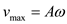Since, the maximum speed of the particle is directly proportional to the amplitude of the particle. Therefore, if the amplitude of the particle is doubled then the speed of the particle will also be doubled.

Tags
Choose question tagWhen a particle in a horizontal SHM is at the equilibrium position, the kinetic energy of the system is (a) zero, (b) at a maximum, (c) half the maximum value, (d) none of the preceding.
Free

A particle exhibits horizontal simple harmonic motion with amplitude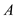; the total energy of the particle is equal to kinetic and potential energies of the particle.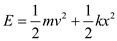Here,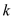is force constant or spring constant,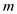and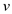are the mass and speed of the particle and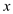is the position of the particle.
(a)
When the oscillating particle passes through its equilibrium position, then in that case the value ofis zero and thus its potential energy is zero. At that instant, the total energy is equal to the kinetic energy and the object is traveling at its maximum speed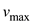.
Hence, total energy of an object in simple harmonic motion of a spring can be calculated as follows:In this case, at equilibrium position only kinetic energy is available as whole potential energy is converted into kinetic energy. Thus, at equilibrium position in a horizontal SHM, the kinetic energy of the system is at maximum.
In option (a), at equilibrium position, the kinetic energy of the system is mentioned as zero but as explained above, the kinetic energy of the system is calculated to be maximum at equilibrium position.
Hence, option (a) is incorrect.
(b)
In option (b), at equilibrium position, the kinetic energy of the system is mentioned to be at a maximum and in the section (a) above also, it is calculated that the kinetic energy of the system is maximum at equilibrium position.
Hence, option (b) is correct.
(c)
In option (c), at equilibrium position, the kinetic energy of the system is mentioned as half the maximum value but in the section (a), it is calculated that the kinetic energy of the system at equilibrium position is maximum and its value is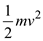.
Hence, option (c) is incorrect.
(d)
In option (d), it is mentioned in the problem that none of the above parts (a), (b) and (c) are correct but as explained above, the section (b) is correct.
Hence, option (d) is incorrect.

Tags
Choose question tagIf the frequency of a system in SHM is doubled, the period of the system is (a) doubled, (b) halved, (c) four times as large, (d) one-quarter as large.
Tags
Choose question tagIf it takes a particle in SHM 0.50 s to travel from the equilibrium position to the maximum displacement (amplitude), what is the period of oscillation
Essay
Tags
Choose question tagFor the SHM equation y = A sin[(200 rad/s) t ], the frequency of oscillation, f , is (a) 50 Hz, (b) 100 Hz, (c) 200 Hz, (d) 200 Hz.
Tags
Choose question tagHow does the speed of a mass in SHM change as the mass leaves its equilibrium position Explain.
Essay
Tags
Choose question tagA particle oscillates in SHM with an amplitude A. What is the total distance (in terms of A ) the particle travels in three periods
Essay
Tags
Choose question tagA tennis player uses a racket to bounce a ball up and down with a constant period. Is this a simple harmonic motion Explain.
Essay
Tags
Choose question tagAn object of mass 1.0 kg is attached to a spring with spring constant 15 N/m. If the object has a maximum speed of 0.50 m/s, what is the amplitude of oscillation
Essay
Tags
Choose question tagFor a particle in SHM, the force on it ( F ) and its displacement from its equilibrium position ( x ) are (a) in the same direction, (b) opposite in direction, (c) perpendicular to each other, (d) none of the preceding.
Tags
Choose question tagIf a mass-spring system were taken to the Moon, would the period of the system change How about the period of a pendulum taken to the Moon Explain.
Essay
Tags
Choose question tagIf the length of a pendulum is doubled, what is the ratio of the new period to the old one
Essay
Tags
Choose question tagThe equation of motion for a particle in SHM (a) is a sine or cosine function, (b) is a tangent or cotangent function, (c) could be any mathematical function, (d) gives the velocity of the particle as a function of time.
Tags
Choose question tagIf you want to increase the frequency of vibration of a mass-spring system, would you increase or decrease the mass Explain.
Essay
Tags
Choose question tagA 0.75-kg object oscillating on a spring completes a cycle every 0.50 s. What is the frequency of this oscillation
Essay
Tags
Choose question tagThe frequency of a simple harmonic oscillator is doubled from 0.25 Hz to 0.50 Hz. What is the change in its period
Essay
Tags
Choose question tagThe maximum kinetic energy of a mass-spring system in SHM is equal to (a) A , (b) A 2 , (c) kA , (d) kA 2 /2.
Tags
Choose question tagA mass-spring system in SHM has an amplitude A and period T. How long does the mass take to travel a distance A How about 2 A
Essay